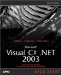Creating Static Properties

Like fields, properties can also be static, which means you don't need an object to use them. As an example, we'll convert the static field numberOfObjects in the static fields example (see Listing 3.7) into a property of the same name . That's easy enoughall we have to do is to implement the new property like this (note that the value of the property is stored in a private field, which must be static so we can access it from the static property):

public class CountedClass {  private static int number = 0;  public CountedClass()   {     number++;   }  public static int NumberOfObjects   {   get   {   return number;   }   set   {   number = value;   }   }  }

Now we can use this new static property as we used the static field earlier, as you see in ch03_09.cs, Listing 3.9.

Listing 3.9 Creating a Static Property (ch03_09.cs)
class ch03_09 {   static void Main()   {  System.Console.WriteLine("Number of objects: {0}",   CountedClass.NumberOfObjects);   CountedClass object1 = new CountedClass();   System.Console.WriteLine("Number of objects: {0}",   CountedClass.NumberOfObjects);   CountedClass object2 = new CountedClass();   System.Console.WriteLine("Number of objects: {0}",   CountedClass.NumberOfObjects);   CountedClass object3 = new CountedClass();   System.Console.WriteLine("Number of objects: {0}",   CountedClass.NumberOfObjects);  } } public class CountedClass {   private static int number = 0;   public CountedClass()   {     number++;   }   public static int NumberOfObjects   {    get    {      return number;    }    set    {      number = value;    }   } }Microsoft Visual C#.NET 2003 Kick Start
ISBN: 0672325470
EAN: 2147483647
Year: 2002
Pages: 181

Similar book on Amazon